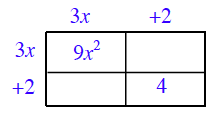### Home > PC3 > Chapter 1 > Lesson 1.1.3 > Problem1-28

1-28.

Is the equation $(3x+2)^2=9x^2+4$ always, sometimes, or never true? Explain.

Complete the generic rectangle below. What is missing?The equation will be true if $x$ is a certain value. What is this value?